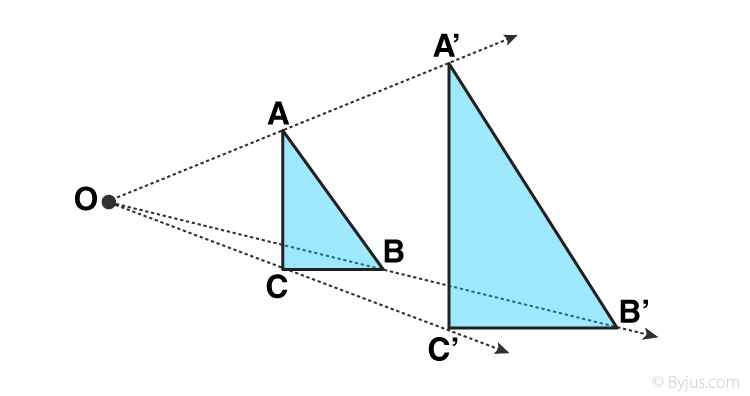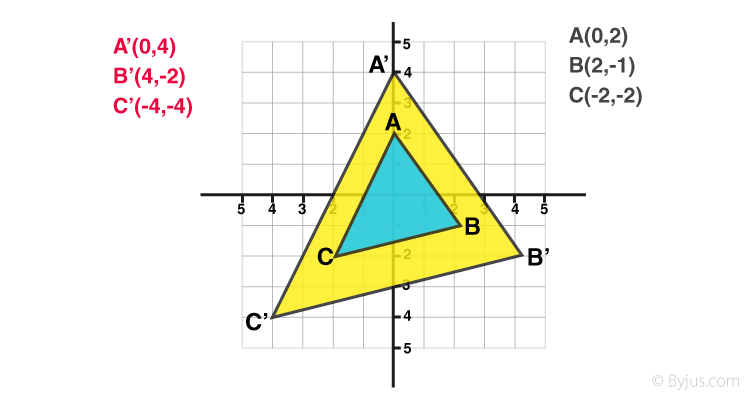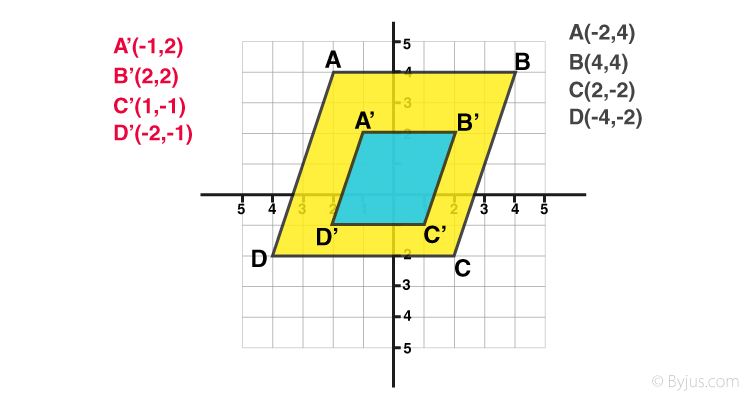# Dilation

Dilation is a process of changing the size of an object or shape by decreasing or increasing its dimensions by some scaling factors. For example, a circle with radius 10 unit is reduced to a circle of radius 5 unit. The application of this method is used in photography, arts and crafts, to create logos, etc. In Geometry, there are four basic types of transformations. They are:

• Rotation
• Translation
• Reflection
• Resizing or Dilation

In this article, let us discuss one of the transformation types called “Dilation” in detail along with the definition, scale factor, properties, and examples.

## Dilation Meaning in Math

Dilation is a transformation, which is used to resize the object. Dilation is used to make the objects larger or smaller. This transformation produces an image that is the same as the original shape. But there is a difference in the size of the shape. A dilation should either stretch or shrink the original shape. This transformation is expressed by the term “scale factor.”

• If a dilation creates a larger image, then it is known as enlargement.
• If a dilation creates a smaller image, then it is known as reduction.In the above figure, we can see, the triangle ACB is transformed into a bigger triangle, i.e., A’B’C’ after the dilation process. Hence, it is the case of enlargement of the size of an object or shape.

### Dilation with Scale Factor

Scale Factor is defined as the ratio of the size of the new image to the size of the old image. The center of dilation is a fixed point in the plane. Based on the scale factor and the center of dilation, the dilation transformation is defined.

• If the scale factor is more than 1, then the image stretches.
• If the scale factor is between 0 and 1, then the image shrinks.
• If the scale factor is 1, then the original image and the image produced are congruent.

### Properties of Dilation

Some features of shapes that remain unchanged during the dilation transformations are:

• Each angle of the figure is the same
• Midpoints of the sides of the figure remain the same as the midpoint of the dilated shape
• Parallel and perpendicular lines in the figure remain the same as the parallel and perpendicular lines of the dilated figure
• The images remain the same

The only change in the dilation process is that the distance between the points changes. It means that the length of the sides of the original image and the dilated image may vary. There are two types of dilation processes. They are:

Horizontal Dilation

The dilation transformation of a function y=f(x) is dilated horizontally by a scale factor C is

Y = f(Cx)

This kind of transformation is called a horizontal dilation.

Vertical Dilation

The dilation transformation of a function y=f(x) is dilated vertically by a scale factor C is

Y = C * f(x)

This kind of transformation is called a Vertical dilation.

### Dilation Examples

Dilation Scale Factor 2:Let the origin (0, 0) be the center of dilation in the coordinate plane.

Let ABC be a triangle in the coordinate plane. The points in the coordinate planes are A(0, 2), B(2, 1), C(-2, -2).

If the scale factor is 2, then every coordinate point of the original triangle is multiplied by the scale factor 2.

Therefore, the dilated triangle will be A’B’C’ and the coordinate points obtained are A’(0, 4), B’(4, 2), C’(-4, -4).

Dilation with scale factor 2, then multiply by 2.

(x, y) → (2x, 2y)

Dilation Scale Factor ½:Assume that, the origin (0, 0) be the center of dilation in the coordinate plane.

Let ABCD be a quadrilateral in the coordinate plane. The points in the coordinate planes are A(-2, 4), B(4, 4), C(2, -2), D(-4, -2)

If the scale factor is 1/2, then every coordinate point of the original triangle is multiplied by the scale factor 1/2.

Therefore, the dilated triangle will be A’B’C’D’ and the coordinate points obtained are A’(-1, 2), B’(2, 2), C’(1, -1), D’(-2, -1)

Dilation with scale factor 1/2, then multiply by 1/2.

(x, y) → (1/2x, 1/2y)

For more interesting concepts on Maths, download BYJU’S – The Learning App and also explore videos to learn with ease.

 Related Links Transformations Rotation Coordinate Geometry 2D Shapes